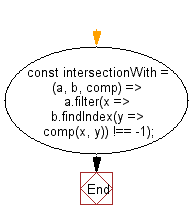# JavaScript: Get a list of elements that exist in both arrays, using a provided comparator function

## JavaScript fundamental (ES6 Syntax): Exercise-209 with Solution

Write a JavaScript program to get a list of elements in both arrays, using a provided comparator function.

• Use Array.prototype.filter() and Array.prototype.findIndex() in combination with the provided comparator to determine intersecting values.

Sample Solution:

JavaScript Code:

``````//#Source https://bit.ly/2neWfJ2
const intersectionWith = (a, b, comp) => a.filter(x => b.findIndex(y => comp(x, y)) !== -1);
console.log(intersectionWith([1, 1.2, 1.5, 3, 0], [1.9, 3, 0, 3.9], (a, b) => Math.round(a) === Math.round(b)));
```
```

Sample Output:

```[1.5,3,0]
```

Flowchart:Live Demo:

See the Pen javascript-basic-exercise-209-1 by w3resource (@w3resource) on CodePen.

Improve this sample solution and post your code through Disqus

What is the difficulty level of this exercise?

Test your Programming skills with w3resource's quiz.

﻿